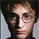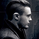22306 lượt xem
This simple indicator gives you a lot of useful information - when to enter, when to exit
and how to reduce risks by entering a trade on a double confirmed signal.
You can use in the xPrice any series: Open, High, Low, Close, HL2 , HLC3, OHLC4 and ect...
```////////////////////////////////////////////////////////////
//  Copyright by HPotter v1.0 25/07/2014
// This simple indicator gives you a lot of useful information - when to enter, when to exit
// and how to reduce risks by entering a trade on a double confirmed signal.
// You can use in the xPrice any series: Open, High, Low, Close, HL2, HLC3, OHLC4 and ect...
////////////////////////////////////////////////////////////
study(title="FX Sniper:  T3-CCI Strategy", shorttitle="T3-CCI")
CCI_Period = input(14, minval=1)
T3_Period = input(5, minval=1)
b = input(0.618)
hline(0, color=purple, linestyle=line)
xPrice = close
b2 = b*b
b3 = b2*b
c1 = -b3
c2 = (3*(b2 + b3))
c3 = -3*(2*b2 + b + b3)
c4 = (1 + 3*b + b3 + 3*b2)
nn = iff(T3_Period < 1, 1, T3_Period)
nr = 1 + 0.5*(nn - 1)
w1 = 2 / (nr + 1)
w2 = 1 - w1
xcci = cci(xPrice, CCI_Period)
e1 = w1*xcci + w2*nz(e1)
e2 = w1*e1 + w2*nz(e2)
e3 = w1*e2 + w2*nz(e3)
e4 = w1*e3 + w2*nz(e4)
e5 = w1*e4 + w2*nz(e5)
e6 = w1*e5 + w2*nz(e6)
xccir = c1*e6 + c2*e5 + c3*e4 + c4*e3
cciHcolor = iff(xccir >= 0 , green,
iff(xccir < 0, red, black))
pos = iff(xccir > 0, 1,
iff(xccir < 0, -1, nz(pos, 0)))
barcolor(pos == -1 ? red: pos == 1 ? green : blue )
plot(xccir, color=blue, title="T3-CCI")
plot(xccir, color=cciHcolor, title="CCIH", style = histogram)```

## Bình luậnHi HPotter,
First of all thanks for the strategy, it is really nice and useful.
I wanted to ask you if you have the same issue I'm having: I sometimes get double alerts on different candles. So, for example, if I entered short, maybe after a candle or two the wave would again "touch" the trend line and consider itself as a crossing down, triggering a second alert.

Is there any way to avoid this?

Thanks!
Phản hồigxyzod
@gxyzod, It may be problem to work realtime. If you try to set xPrice = close it can be fix it.
Phản hồiCailz
@Cailz, Press add to favorite and looking for in the your scripts dialog.
Phản hồiLucaaa
@Lucaaa, Do not know. It should be tested for each tf and symbol.
Phản hồiGood afternoon! Thanks for the great indicator! Is it possible to create alerts when changing a trend? Thank you!
Phản hồi